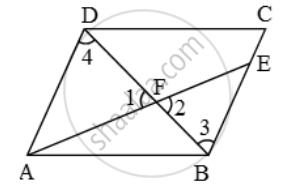# The diagonal BD of a parallelogram ABCD intersects the segment AE at the point F, where E is any point on the side BC. Prove that DF × EF = FB × FA - Mathematics

Sum

The diagonal BD of a parallelogram ABCD intersects the segment AE at the point F, where E is any point on the side BC. Prove that DF × EF = FB × FA

#### Solution

In ∆AFD and ∆BFE, we have

∠1 = ∠2 [Vertically opposite angles]

∠3 = ∠4 [Alternate angles]So, by AA-criterion of similarity, we have

∆FBE ~ ∆FDA

\Rightarrow \frac{FB}{FD}=\frac{FD}{FA}

\Rightarrow \frac{FB}{DF}=\frac{EF}{FA}

⇒ DF × EF = FB × FA

Concept: Similarity
Is there an error in this question or solution?